# 6 Making Tables

 6 Making Tables
We’ve seen a few ways to make lists in the Wolfram Language. You can just type them in. You can use Range. And you can use functions like IntegerDigits. One of the most common and flexible ways to make lists is with the function Table.
In its very simplest form, Table makes a list with a single element repeated some specified number of times.
Make a list that consists of 5 repeated 10 times:
Table[5, 10]

This makes a list with x repeated 10 times:
Table[x, 10]

You can repeat lists too:
Table[{1, 2}, 10]

Or, actually, anything; here’s a list of 3 identical pie charts:
Table[PieChart[{1, 1, 1}], 3]

But what if we want to make a table where the elements aren’t identical? We can do that by introducing a variable, and then iterating over that variable.
Iterate over n to make a list where n goes up to 5:
Table[a[n], {n, 5}]

Here’s how this works. To make the first element of the list, n is taken to be 1, so a[n] is a. To make the second element, n is taken to be 2, so a[n] is a, and so on. n is called a variable because it’s varying as we make the different elements of the list.
Make a table that gives the value of n+1 when n goes from 1 to 10:
Table[n + 1, {n, 10}]

Table[n^2, {n, 10}]

With Table, you can make tables of anything.
Here’s a table of successively longer lists produced by Range:
Table[Range[n], {n, 5}]

Here each of the lists produced is shown as a column:
Table[Column[Range[n]], {n, 8}]

Here’s a table of plots of successively longer lists of values:
Table[ListPlot[Range[10*n]], {n, 3}]

Here are pie charts with successively more segments:
Table[PieChart[Table[1, n]], {n, 5}]

Table[2^expt, {expt, 10}]

Here we’re using x as the variable name, and it happens to appear several times:
Table[{x, x + 1, x^2}, {x, 5}]

In Table[f[n],{n,5}], n takes on values 1, 2, 3, 4, 5. Table[f[n],{n,3,5}] says to start at 3 instead: 3, 4, 5.
This generates a table with n going from 1 to 10:
Table[f[n], {n, 10}]

This generates a table with n going from 4 to 10:
Table[f[n], {n, 4, 10}]

This makes n go from 4 to 10 in steps of 2:
Table[f[n], {n, 4, 10, 2}]

The Wolfram Language emphasizes consistency, so for example Range is set up to deal with starting points and steps just like Table.
Generate the range of numbers 4 to 10:
Range[4, 10]

Generate the range of numbers 4 to 10 going in steps of 2:
Range[4, 10, 2]

Go from 0 to 1 in steps of 0.1:
Range[0, 1, 0.1]

Generate a table and plot it:
ListPlot[Table[x - x^2, {x, 0, 1, .02}]]

Get the same result by doing arithmetic with the range of values:
ListPlot[Range[0, 1, .02] - Range[0, 1, .02]^2]

Table always separately computes each entry in the list it generatesand you can see this if you use RandomInteger in Table.
This generates 20 independent random integers with size up to 10:
Table[RandomInteger, 20]

RandomInteger can actually also generate the list directly.
This again generates 20 random integers with size up to 10:
RandomInteger[10, 20]

6.1Make a list in which the number 1000 is repeated 5 times. »
Expected output:
 Out[]=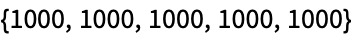Answer & check your solution
6.2Make a table of the values of n^3 for n from 10 to 20. »
Expected output:
 Out[]=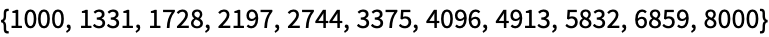Answer & check your solution
6.3Make a number line plot of the first 20 squares. »
Expected output:
 Out[]=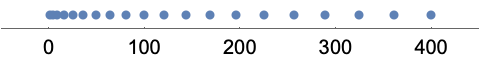Answer & check your solution
6.4Make a list of the even numbers (2, 4, 6, ...) up to 20. »
Expected output:
 Out[]=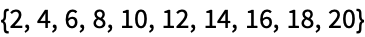Answer & check your solution
6.5Use Table to get the same result as Range»
Expected output:
 Out[]=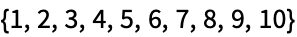Answer & check your solution
6.6Make a bar chart of the first 10 squares. »
Expected output:
 Out[]=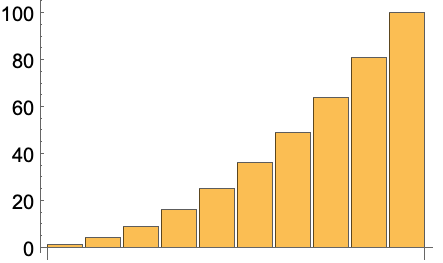Answer & check your solution
6.7Make a table of lists of digits for the first 10 squares. »
Expected output:
 Out[]=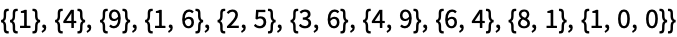Answer & check your solution
6.8Make a list line plot of the length of the sequence of digits for each of the first 100 squares. »
Expected output:
 Out[]=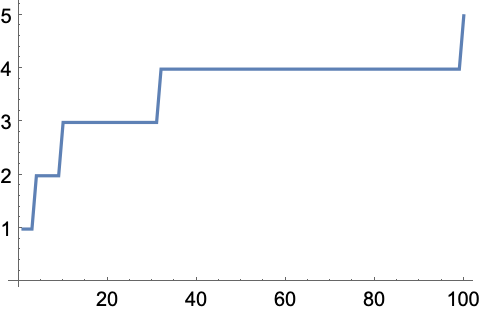Answer & check your solution
6.9Make a table of the first digit of the first 20 squares. »
Expected output:
 Out[]=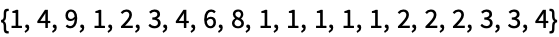Answer & check your solution
6.10Make a list line plot of the first digits of the first 100 squares. »
Expected output:
 Out[]=Answer & check your solution
+6.1Make a list of the differences between n^3 and n^2 with n up to 10. »
Expected output:
 Out[]=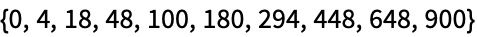Answer & check your solution
+6.2Make a list of the odd numbers (1, 3, 5, ...) up to 100. »
Expected output:
 Out[]=Answer & check your solution
+6.3Make a list of the squares of even numbers up to 100. »
Expected output:
 Out[]=Answer & check your solution
+6.4Create the list {-3, -2, -1, 0, 1, 2} using Range»
Expected output:
 Out[]=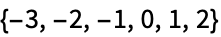Answer & check your solution
+6.5Make a list for numbers n up to 20 in which each element is a column of the values of n, n^2 and n^3»
Expected output:
 Out[]=Answer & check your solution
+6.6Make a list line plot of the last digits of the first 100 squares. »
Expected output:
 Out[]=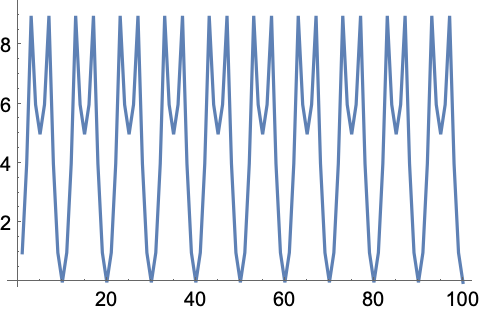Answer & check your solution
+6.7Make a list line plot of the first digit of the first 100 multiples of 3. »
Expected output:
 Out[]=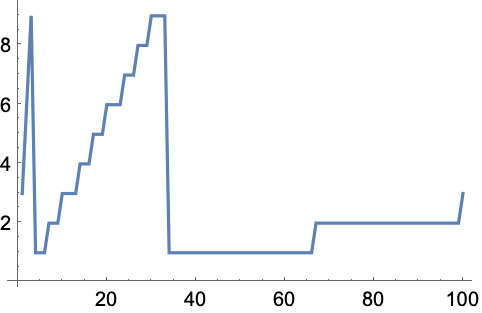Answer & check your solution
+6.8Make a list line plot of the total of the digits for each number up to 200. »
Expected output:
 Out[]=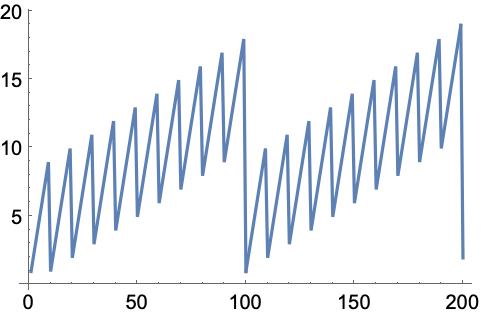Answer & check your solution
+6.9Make a list line plot of the total of the digits for each of the first 100 squares. »
Expected output:
 Out[]=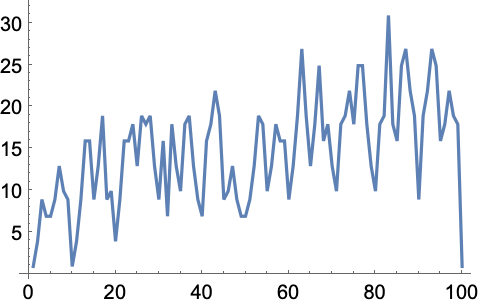Answer & check your solution
+6.10Make a number line plot of the numbers 1/n with n from 1 to 20. »
Expected output:
 Out[]=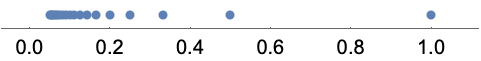Answer & check your solution
+6.11Make a line plot of a list of 100 random integers where the nth integer is between 0 and n»
Sample expected output:
 Out[]=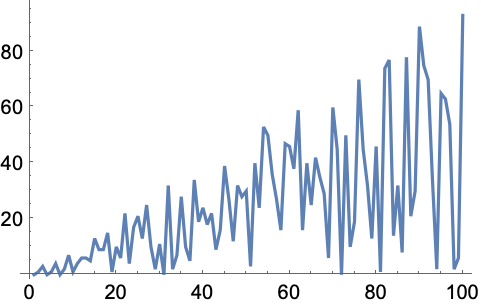Answer & check your solution
A list is always a way of collecting things together. Here what it’s collecting is the variable n and its range 5. In the Wolfram Language, this kind of use of a list is called an iterator specification.
Why is the { ...} (list) in Table[n^2, {n, 5}] needed?
So one can easily generalize to multidimensional arrays, like Table[x^2-y^2, {x, 5}, {y, 5}].
What are the constraints on the names of variables?
They can be any sequence of letters or numbers, but they can’t start with a number, andto avoid possible confusion with built-in Wolfram Language functionsthey shouldn’t start with a capital letter.
Why do you have to name a variable if the name never matters?
Good question! In Section 26, we’ll see how to avoid having named variables. It’s very elegant, but it’s a little more abstract than what we’re doing with Table here.
Can Range deal with negative numbers?
Yes. Range[-2, 2] gives {-2, -1, 0, 1, 2}. Range[2, -2] gives {}, but Range[2, -2, -1] gives {2, 1, 0, -1, -2}.